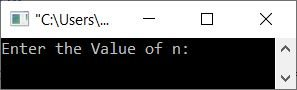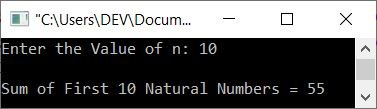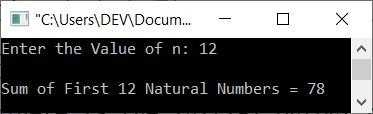# C++ Program to Find and Print the Sum of First n Natural Numbers

This article is created to provide some programs in C++ that find and print the sum of the first n natural numbers. The value n must be entered by the user at run-time. The program is created in the following ways:

Note: A natural number starts with 1 and continues like 1, 2, 3,...

## Find the sum of first n natural numbers using the while loop

The question is: write a program in C++ that receives the value of n and prints the sum of the first n natural numbers. Here is its answer:

```#include<iostream>
using namespace std;

int main()
{
int n, natural=1, sum=0;
cout<<"Enter the Value of n: ";
cin>>n;
while(natural<=n)
{
sum = sum+natural;
natural++;
}
cout<<"\nSum of First "<<n<<" Natural Numbers = "<<sum;
cout<<endl;
return 0;
}```

Here is the initial output produced by the above C++ program on finding and printing the sum of n natural numbers:Now supply the number, say 10, as the value of n and press the ENTER key to find the sum of the first 10 natural numbers, as shown in the snapshot given below:## Find the sum of first n natural numbers using the for loop

This program is created using the for loop instead of while.

```#include<iostream>
using namespace std;

int main()
{
int n, natural, sum=0;
cout<<"Enter the Value of n: ";
cin>>n;
for(natural=1; natural<=n; natural++)
sum = sum+natural;
cout<<"\nSum of First "<<n<<" Natural Numbers = "<<sum;
cout<<endl;
return 0;
}```

Here is its sample run with user input: 12.## Find the sum of first n natural numbers using function

This is the last program created using a user-defined function named myfun() that takes an integer, say n, as its argument and then finds and returns the sum of the first n natural numbers.

```#include<iostream>
using namespace std;

int myfun(int);
int main()
{
int n, sum;
cout<<"Enter the Value of n: ";
cin>>n;
sum = myfun(n);
cout<<"\nSum of First "<<n<<" Natural Numbers = "<<sum;
cout<<endl;
return 0;
}
int myfun(int n)
{
int natural, sum=0;
for(natural=1; natural<=n; natural++)
sum += natural;
return sum;
}```

C++ Quiz

« Previous Program Next Program »